## Main

The recent discovery of the three-dimensional (3D) Dirac semimetals Cd3As2 and Na3Bi (refs 3,4,5,6) has enabled experimental studies of the quantum dynamics of relativistic field theory in condensed matter systems. The relativistic theory of charged chiral fermions in three spatial dimensions possesses the so-called chiral anomaly1,2—non-conservation of chiral charge induced by external gauge fields with non-trivial topology, for example, by parallel electric and magnetic fields. The existence of chiral quasiparticles in Dirac and Weyl semimetals opens the possibility to observe the effects of the chiral anomaly7. Of particular interest is the chiral magnetic effect (CME; ref. 8)—the generation of electric current in an external magnetic field induced by the chirality imbalance9.

This phenomenon is at present under intense study in relativistic heavy ion collisions at the Relativistic Heavy Ion Collider (RHIC) at BNL and at the Large Hadron Collider (LHC) at CERN, where it was predicted10 to induce fluctuations in hadron charge asymmetry with respect to the reaction plane. The experimental data from the STAR (ref. 11) Collaboration at RHIC and the ALICE (ref. 12) Collaboration at LHC indicate the fluctuations are consistent with the theoretical expectations. Closely related phenomena are expected to play an important role in the Early Universe, possibly causing the generation of primordial magnetic fields13,14,15,16,17. However, the interpretation in all these cases is under debate owing to lack of control over the chirality imbalance produced.

The most prominent signature of the CME in Dirac systems in parallel electric and magnetic fields is a positive contribution to the conductivity that has a quadratic dependence on magnetic field8,18,19. This is because the CME current is proportional to the product of the chirality imbalance and the magnetic field, and the chirality imbalance in Dirac systems is generated dynamically through the anomaly with a rate that is proportional to the product of electric and magnetic fields. As a result, the longitudinal magnetoresistance becomes negative18,19.

Let us explain how this mechanism works in Dirac semimetals in more detail. In the absence of external fields, each Dirac point initially contains left- and right-handed fermions with equal chemical potentials, μL = μR = 0. If the energy degeneracy between the left- and right-handed fermions gets broken, we can parameterize it by introducing the chiral chemical potential μ5 ≡ (μRμL)/2. The corresponding density of chiral charge is then given by8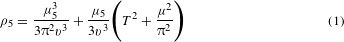where μ = (μR + μL)/2 and T are the chemical potential and the temperature, and v is the Fermi velocity.

The chiral anomaly of quantum electrodynamics dictates that the parallel external electric and magnetic fields generate the chiral charge with a rate given by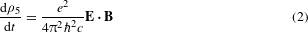However, the left- and right-handed fermions in Dirac semimetals can mix through chirality-changing scattering, and this process will deplete the amount of chiral charge that can be produced. Denoting the chirality-changing scattering time by τV, we thus get the equation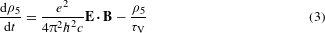The solution of equation (3) at t τV is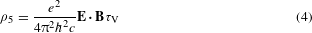According to equation (1), this leads to a non-zero chiral chemical potential μ5 (we assume that μ5 μ, T):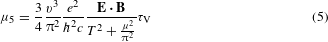On the lowest Landau level, the spins of positive (negative) chiral fermions are parallel (anti-parallel) to the external magnetic field. Therefore, for a positive fermion to be right-handed (that is, have a positive projection of spin on momentum) it means moving along the magnetic field, whereas for a negative fermion it means moving against the magnetic field. The left-handed fermions will move in the opposite directions, so there will normally be no charge separation. However, if the densities of the right- and left-handed fermions are different, the currents of positive and negative charges do not compensate each other, and the system develops a net electric current—this is the CME. The corresponding current can be computed8 by field-theoretical methods and is given by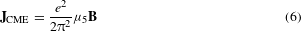The formulae (6) and (5) yield the final expression for the CME current: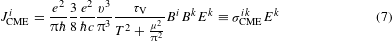We see that the CME is described by the conductivity tensor σCMEik BiBk. When the electric and magnetic fields are parallel, the CME conductivity is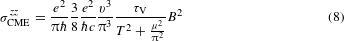Because the CME current is directed along the electric field, it will affect the measured conductivity, as the total current will be the sum of the Ohmic and CME currents: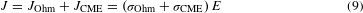where σCMEσCMEzz. If the electric and magnetic fields are parallel (θ = 0), there is no conventional contribution to magnetoresistance induced by the Lorentz force. The magnetoconductance (8) has a characteristic quadratic dependence on magnetic field. It is precisely this contribution to magnetoconductance with a quadratic dependence on magnetic field that we have unambiguously observed in ZrTe5.

ZrTe5 is a material that crystallizes in the layered orthorhombic crystal structure, with prismatic ZrTe6 chains running along the crystallographic a-axis and linked along the c-axis by zigzag chains of Te atoms to form two-dimensional (2D) layers, stacked along the b-axis into a crystal. This material is known for its large thermoelectric power, resistivity anomaly20 and large positive magnetoresistance21. It shows a semimetallic electronic structure with extremely small and light ellipsoidal Fermi surface(s), centred at the centre (Γ point) of the bulk Brillouin zone (BZ). The calculations predict a small direct gap at Γ(50 meV), but previous transport studies show semimetallic behaviour, with quantum oscillations indicating a tiny but finite Fermi surface22,23,24,25. Quantum oscillations show that the effective mass in the chain direction (ma/me0.03) is comparable to that in a prototypical 3D Dirac semimetal, Cd3As2 (refs 24,26).

Figure 1a shows the temperature dependence of resistivity along the chain direction (a) in a magnetic field perpendicular to the ac plane. The zero-field transport shows a characteristic peak in resistivity at T 60 K, significantly lower than in earlier studies, probably due to a much lower impurity concentration in our samples20. In B || b, the peak shifts to higher temperatures and we observe a very large positive magnetoresistance in the whole temperature range, consistent with previous studies21. The inset shows the resistivity with the magnetic field applied along the three major crystal axes.

Figure 1c, d shows the magnetoresistance measured at 20 K for several angles of the applied magnetic field with respect to the current along the chain direction. The angle rotates from the b-axis to the a-axis, so that at ϕ = 90° the field is parallel to the current (B || a)—the so-called Lorentz-force-free configuration. When the magnetic field is aligned along the b-axis (ϕ = 0°), the magnetoresistance is positive and quadratic in low fields, and tends to saturate in high fields. The similar angular dependence is also observed for the field in the ca plane. When the magnetic field is rotated away from the b- or c-axis, the positive magnetoresistance drops with cosφ, as expected for the Lorentz force component. However, in the Lorentz-force-free configuration (B || a), we see a large negative magnetoresistance, a clear indication of CME in this material.

Figure 2 shows the magnetoresistance at various temperatures in a magnetic field parallel to the current. At elevated temperatures, T ≥ 110 K, the ρ versus B curves show a small upward curvature, a contribution from an inevitable perpendicular field component due to imperfect alignment between the current and the magnetic field. In fact, the small perpendicular field contribution to the observed resistivity can be fitted with a simple quadratic term (Supplementary Information and Supplementary Fig. 1). This term is treated as a background and subtracted from the parallel field component for all magnetoresistance curves recorded at T ≤ 100 K.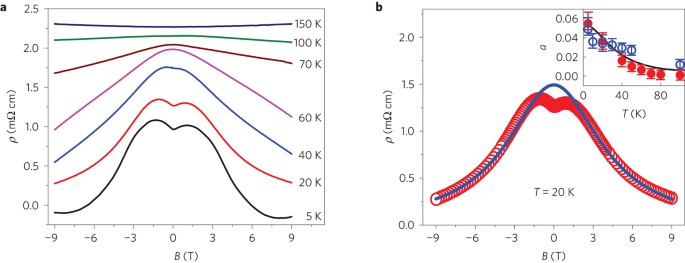Figure 2: Magnetoresistance in magnetic fields parallel to the current (B || a) in ZrTe5.

A negative magnetoresistance is observed for T ≤ 100 K, increasing in magnitude as temperature decreases. We found that the magnetic field dependence of the negative magnetoresistance can be nicely fitted with the CME contribution to the electrical conductivity, given by σCME = σ0 + a(T)B2, where σ0 represents the zero-field conductivity. The fitting is illustrated in Fig. 2b for T = 20 K, with an excellent agreement between the data and the CME fitting curve. At 4 T, the CME conductivity is about the same as the zero-field conductivity. At 9 T, the total magnetoconductivity increases by 400% over the zero-field value as a result of the CME contribution. This results in a negative magnetoresistance that is much stronger than any conventional magnetoresistance reported at an equivalent magnetic field in a non-magnetic material. To demonstrate that the effect is not limited to any specific crystal direction, but locked only to the current direction, we have also performed magnetoresistance measurements with the current still in the ac plane, but 18° off the chain direction (a-axis). In this direction, we observe the same B2 dependence of CME when the magnetic field is parallel to the current.

To obtain a numerical theoretical estimate for CME, we take T = 20 K, ρOhm 1.2 mΩ cm indicated by our measurements at B = 0, and assume that the rate of chirality-changing transitions τV−1 = Δ/. Δ 50 meV and μ 9 meV is determined from the fit to the data. The ARPES data indicate that v 1/300 c. Evaluating equation (8), we reproduce the correct order of magnitude of the coefficient a(T) that we used to fit the quadratic dependence of magnetoresistance on magnetic field. The inset in Fig. 2b shows the temperature dependence of parameter a(T) for B and E along the a-axis (red solid symbols) and 18° off the a-axis, in the ac plane (open circles), together with the dependence (line) predicted by equation (8) in which the effective value of μ is found to be μ 9 meV.

At very low field, the data show a small cusp-like feature. The origin of this feature is not completely understood, but it probably indicates some form of weak anti-localization (WAL). This, and the large positive magnetoresistance for magnetic field perpendicular to current, seem to be common features in all the materials that were subsequently found to exhibit the CME in parallel fields27,28,29,30,31,32. Although the reason for a simultaneous observation of CME in parallel fields and large magnetoresistance in perpendicular fields is not fully understood yet, we note that the general requirements for the observation of CME—that is, the low carrier concentration and nearly linear bands—seem to be also favourable for the observation of large positive magnetoresistance in perpendicular fields. In these situations, the system is either very close to the quantum limit33 or, if it contains two types of carriers, the charge compensation may lead to a large response in the way that was recently observed in WTe2 (refs 34,35,36).

A necessary requirement for observation of the CME is that a material has a 3D Dirac semimetal-like (zero gap), or semiconductor-like (non-zero gap) electronic structure. Figure 3 shows angle-resolved photoemission spectroscopy (ARPES) data from a freshly cleaved (ac plane) ZrTe5 sample. The states forming the small, hole-like Fermi surface (FS) disperse linearly over a large energy range, both along the chain direction (Fig. 3c) and perpendicular to it (Fig. 3a), indicating a Dirac-like dynamics of carriers for the in-plane propagation. The velocity, or the slope of dispersion, is very large in both the chain direction, va 6.4 eV Å (c/300), and perpendicular to it, vc 4.5 eV Å. If extrapolated to the unoccupied part, the linear approximation for this band would place the Dirac point at 0.2 eV above the EF.

In Fig. 3c, it also seems that the bottom of a tiny electron pocket is enclosed by the dominant hole-cone. However, owing to a sizable quasiparticle scattering, ΓQ = /τQ 100 meV, the states are apparently too broad to completely resolve possible fine features in the low-energy electronic structure, such as the existence of small gaps or changes in the velocity at low energies (E < 50 meV). Nevertheless, the magnitude of CME in ZrTe5 is not particularly sensitive to the fine details in the electronic structure on an energy scale smaller than ΓQ. On the other hand, for the existence of CME, it is crucial that the electronic structure of a material is 3D Dirac-like. Although recent magneto-optical studies have shown that ZrTe5 is indeed a 3D Dirac semimetal37, it has been also suggested that this material might be either a weak or strong topological insulator25. It is therefore important to verify that the states seen in ARPES are bulk states and not topological surface states.

This is done in Fig. 3e–h, which shows the dependence of the states forming the FS on the momentum kb perpendicular to the ac plane. Figure 3e, f shows a clear warping of the Fermi surface and a significant dispersion with kb, indicating the bulk character of the measured states. Unfortunately, because the Dirac point is unoccupied, the ARPES studies cannot provide a complete picture of the electronic structure in its vicinity, and the existence of a small mass gap cannot be excluded. The occupied part of the cone (Fig. 3f–h) indicates that the out-of-plane velocity is an order of magnitude smaller than the in-plane velocity, consistent with ZrTe5 being a highly anisotropic 3D Dirac semimetal.

We note that the possible coexistence of both the hole and electron pockets might be responsible for the large positive magnetoresistance seen in the perpendicular field, as the fine balance between them could lead to a perfect compensation at some temperature34,35,36. We also note that the tiny volume of the FS indicates a very low carrier concentration, 1017–1018 cm−3, consistent with the absence of quantum oscillations in transport measurements in the probed field range.

As noted, the ARPES measurements cannot exclude a possible gap in the otherwise Dirac-like spectrum. The gap Δ will in general induce chirality-changing transitions with a rate Δ/—this is because the corresponding term in the effective Hamiltonian, namely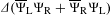, mixes the left and right components of the spinors—but it does not prevent the CME. The ARPES data also indicate that the quantum scattering rate determined from the broadening of quasiparticles is ΓQ = /τQ 100 meV. Chirality conservation in the quasiparticle scattering processes implies that the chirality-changing rate should be only a small fraction of the quantum scattering rate, ΓV = /τV ΓQ. It is therefore reasonable to assume that the measured ΓQ represents the absolute upper limit to the chirality-changing transition rate. The existence of intra-valley transitions does not destroy the CME, although the chirality-flipping transitions do reduce the magnitude of the CME current, as can be seen in our equation (8) for the CME conductivity, which is inversely proportional to the chirality-flipping rate.

We note that equation (9) shows that, in the regime where ρOhm ρCME, the measured magnetoresistivity ρ directly provides the CME resistivity, ρ ρCME. Therefore it is much easier to observe the CME in materials that have a relatively large zero-field resistivity. The zero-field resistivity in ZrTe5 is ρOhm 1.2 mΩ cm at T = 20 K, and according to our theoretical estimates the CME and Ohmic resistivities become equal, ρOhm ρCME, at B 3 T. On the other hand, if a material had a much smaller resistivity, the CME observation would have been more difficult.

The present study can be extended to a broad range of materials as 3D Dirac semimetals often emerge at quantum transitions between conventional (‘trivial’) and topological insulators, and between conventional and topological crystalline insulators. Indications that the CME occurs at the former type of transitions have already been observed in Bi1−xSbx at x 0.03 (ref. 33). In addition, our experimental observation has important implications extending well beyond condensed matter physics.

After submission of our manuscript, we noticed a surging interest in the study of magnetotransport in Dirac-like semimetals, with several recent preprints reporting a negative magnetoresistance as a consequence of the CME. The effect is observed in two types of 3D chiral materials. The first type consists of Dirac semimetals, where the Dirac points for left- and right-handed fermions are not separated in momentum space. These materials are effectively transformed into Weyl semimetals by parallel electric and magnetic fields, with an imbalance between the densities of left- and right-handed fermions induced by the chiral anomaly. Members of that class are ZrTe5, Na3Bi (ref. 27), Cd3As2 (ref. 32) and Bi1−xSbx (x 0.03; ref. 33). In particular, a very detailed transport measurement was performed recently with the Dirac semimetal Na3Bi (ref. 27), with results similar to ours. They observed both a cusp of longitudinal magnetoresistance near zero magnetic field and a negative longitudinal magnetoresistance in stronger fields due to the CME, with a steep dependence on the angle between electric and magnetic fields27. The second type of 3D chiral materials consists of inversion asymmetric Weyl semimetals, such as TaAs (ref. 28), NbAs (ref. 29), NbP (ref. 30) and TaP (ref. 31). In both types of materials, parallel electric and magnetic fields are required to observe the CME.

## Methods

### Crystal growth.

Crystals of ZrTe5 were grown by the flux growth method using Te as flux. High-purity elements (99.99999% Te and 99.9999% Zr) were loaded into a double-walled quartz ampoule and sealed under vacuum. The composition of the Zr–Te melt used for the ZrTe5 growth was Zr0.0025Te0.9975. The materials were first melted at 900 °C in a box furnace and fully rocked for 72 h to achieve a homogeneous mixture. The melt was then slowly cooled and rapidly heated (for re-melting of small size crystals) between 445 and 505 °C for 21 days. The largest single crystals were 0.1 × 0.3 × 20 mm3. Crystals were chemically and structurally characterized by powder X-ray diffraction, scanning electron microscopy with energy dispersive X-ray analysis, and transmission electron microscopy with electron diffraction.

### Transport measurements.

The magnetoresistance of ZrTe5 samples was measured using the four-point probe in-line method in a Quantum Design Physical Property Measurement System (PPMS) equipped with 9 T superconducting magnet. Measurements have been performed with the current along the chain direction (a) and 18° off the chain direction, but still in the ac plane. The off a-axis measurements were extremely difficult, owing to the needle-like aspect of our single crystals and their small size along the c and b-axes. For crystal alignment with the magnetic field, horizontal and vertical sample rotators were used with an angular resolution of 0.1°. Temperature-dependent data were taken from 1.8 to 400 K, at various fields up to 9 T.

### ARPES experiments.

The ARPES measurements were conducted using a Scienta SES2002 analyser at the U13 beam line of the National Synchrotron Light Source at Brookhaven National Laboratory (hv = 21 eV), and at the 12.0.1 beam line of the Advanced Light Source at Lawrence Berkeley National Laboratory (35–80 eV) using a Scienta R 3000 analyzer. The total experimental resolution was 15 meV in energy and ≤0.2° in angle, in both experimental set-ups. The two-dimensional Brillouin zone mapping was accomplished by rotating the sample perpendicularly to the analyser slit, in steps of 1° at hv = 21 eV and 0.5° at higher photon energies. The samples were glued to the holder using a conductive epoxy resin and cleaved at the ac plane in an ultrahigh vacuum (p < 10−8 Pa) just before the measurements. Sample cooling was provided through contact with cryostats filled with liquid helium or liquid nitrogen.

The evolution of electronic structure with photon energy reflects the dispersion along kz (kb in our notation). Even though kz is not conserved in a photoemission process, it can be approximated by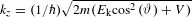, where Ek is the kinetic energy of a photoelectron and V is the inner potential. A set of ARPES spectra taken along ka = 0 in the surface BZ at different photon energies (39 ≤ hv ≤ 79 eV, in 2 eV steps) is converted into a (kc, kb, E) data set by using the inner potential V = 13 eV. The E = 0 slice, representing the FS, is shown in Fig. 3e, and the dispersion of the states at ka = 0, kc = −0.055 Å−1 is shown in Fig. 3f. We note that in this photon energy range, the 9th to the 11th BZs are probed. States localized to the surface (surface states) do not disperse with kz and would form straight vertical streaks in Fig. 3e, if they existed.

By evaluating the volume of the FS from our ARPES measurements, VFS, we can estimate the carrier concentration in our samples, n = 2 VFS/(2π)3, in the low 1018 cm−3 range. Here, we have taken into account the spin degeneracy.